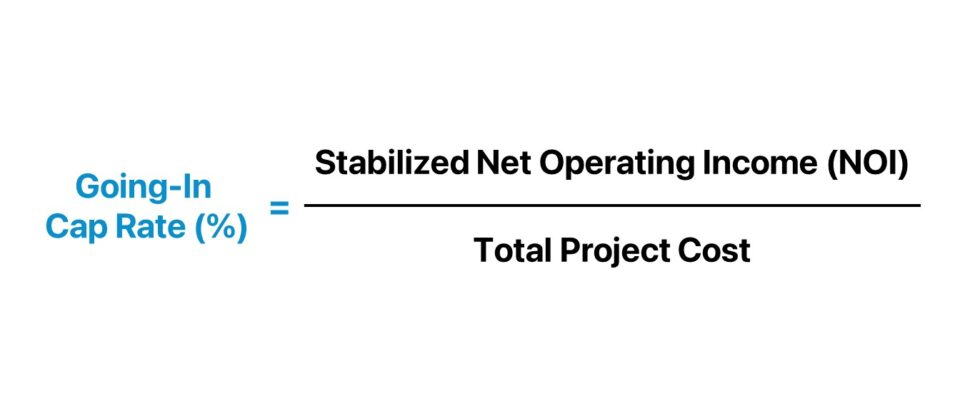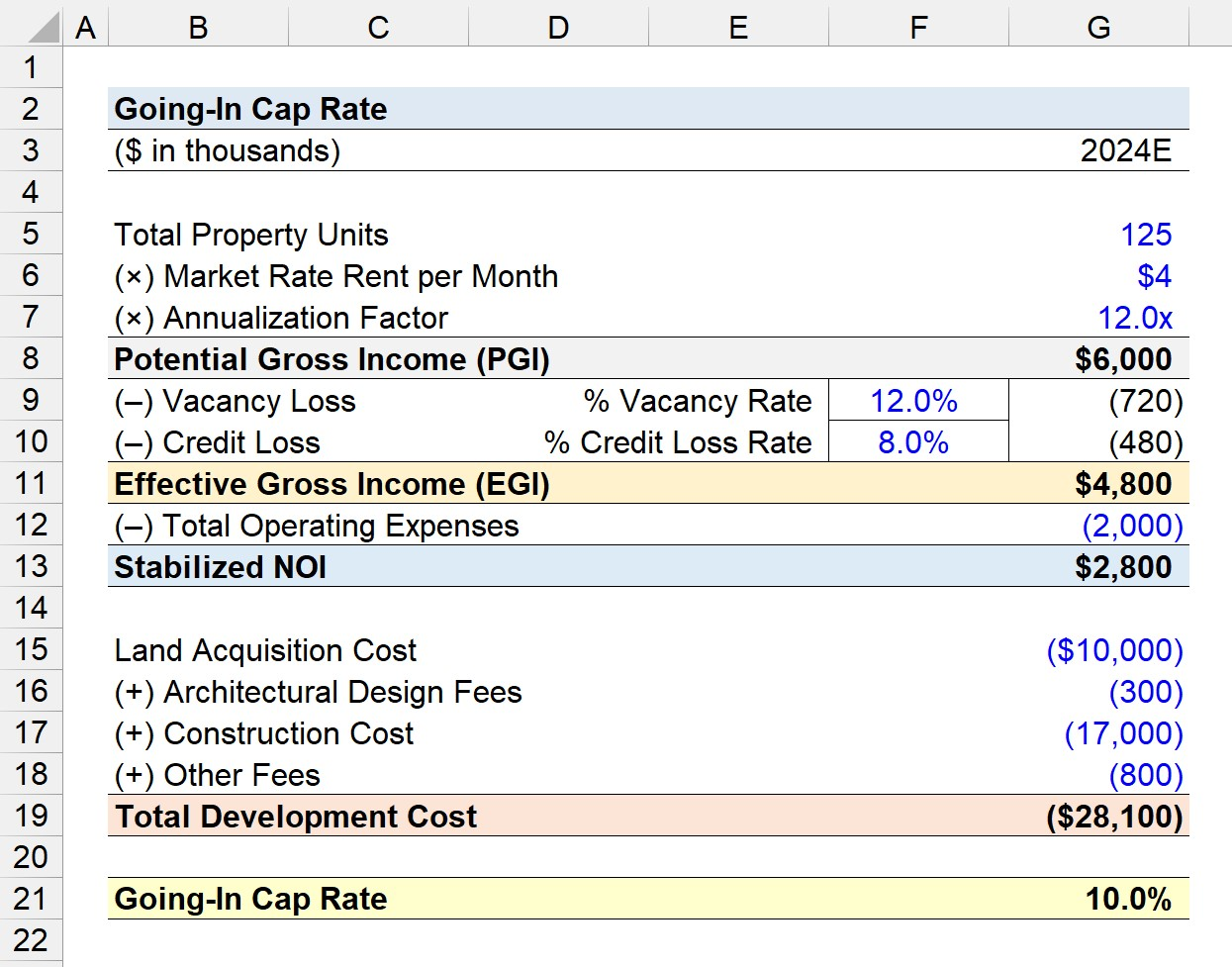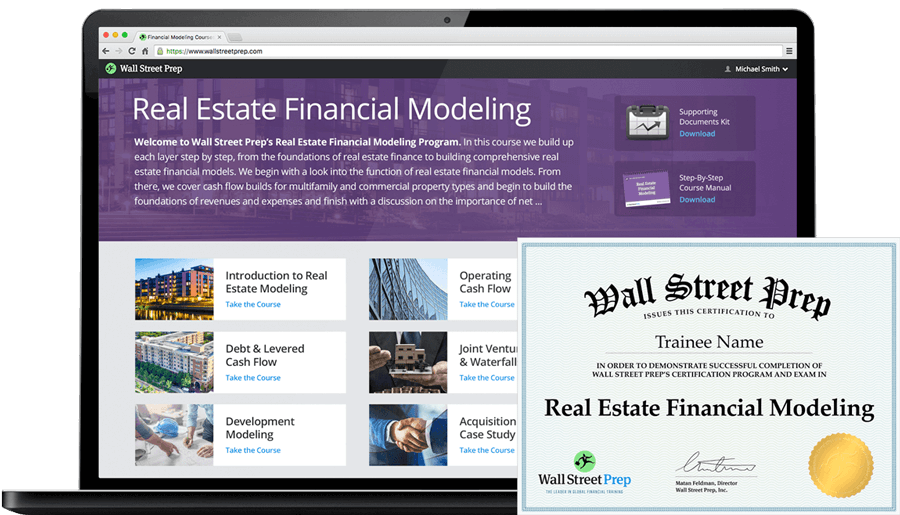Welcome to Wall Street Prep! Use code at checkout for 15% off.# Going-In Cap Rate

Guide to Understanding the Going-In Cap Rate## How to Calculate Going-In Cap Rate?

The “going-in” cap rate is a real estate investing metric wherein the traditional capitalization rate is modified to measure the yield as of the date of property stabilization.

The capitalization rate, or “cap rate” for short, estimates the implied yield earned on an income-generating property investment.

In particular, the use-case of the going-in cap rate is intended for development projects, which involve a period of construction work when there are no tenants (and thus no income).

Once the construction of the property is complete and rental leases are signed with tenants – i.e. the investment property is now fully operational – the property is deemed “stabilized”.

In real estate investing, development projects differ from property acquisitions because in the latter investment type, the acquired property generates cash flow starting immediately from the initial purchase date.

The going-in cap rate is calculated by dividing the stabilized net operating income (NOI) of the property by its total purchase price.

• Stabilized NOI → The pro forma net operating income (NOI) of a property once a state of normalization is reached.
• Total Purchase Price → The total costs incurred by the real estate investors as part of undertaking the property development or acquisition project.

The stabilized net operating income (NOI) is often annualized by multiplying the NOI of the most recent month and multiplying it by 12 or by multiplying the pro forma forward three months NOI and multiplying by 4.

Other adjustments to further normalize the net operating income (NOI) for external factors such as seasonality can also be necessary.

Under the context of a development project – contrary to an acquisition project – the total cost component includes the following:

• Land Purchase Price
• Construction Costs
• Transaction Advisory and Legal Fees (3rd Party Professional Fees)
• Financing Fees (e.g. Origination Fee)

On the other hand, the total purchase cost is simply the denominator for acquisition projects.## Going-In Cap Rate Formula

The going-in cap rate formula is as follows.

Going-In Cap Rate (%) = Stabilized Net Operating Income (NOI) ÷ Total Project Cost

The output will be in decimal format, so it is necessary to then multiply the resulting figure by 100 to convert the going-in cap rate into a percentage.

## Going-In Cap Rate Calculator

We’ll now move to a modeling exercise, which you can access by filling out the form below.#### Get the Excel Template!Submitting...

## 1. Effective Gross Income Calculation (EGI)

Suppose a real estate investor is calculating the going-in cap rate of a development project.

The real estate development project is a residential building anticipated to be complete and reach stabilization in 2024.

The residential building, once construction and repair work is complete, will consist of a total of 125 units, with a market rent of \$4k per month.

Pro Forma 2024E Model Assumptions

• Total Property Units = 125
• Rent per Month, Market Rate = \$4k
• Vacancy Rate (% of PGI) = 12%
• Credit Loss Rate (% of PGI) = 8%

Note that the projected vacancy and credit losses are on the higher end because the building is still in the earlier stages of its lifecycle.

The building will, over time, become more efficient at collecting rent payments from tenants and screening and performing credit analysis on tenants to reduce the number of defaults.

From the property’s potential gross income (PGI), the next step is to deduct the property’s expected vacancy and credit losses.

The resulting metric, the effective gross income (EGI), comes out to \$4.8 million.

• Vacancy Loss = 12% × \$6 million = \$720k
• Credit Loss = 8% × \$6 million = \$480k
• Effective Gross Income (EGI) = \$6 million – \$720k – \$480k = \$4.8 million

## 2. Stabilized Net Operating Income Calculation (NOI)

Our next step involves calculating the stabilized net operating income (NOI) of the residential building.

The stabilized NOI is determined by subtracting the property’s total operating expenses from its effective gross income (EGI).

The total operating expenses expected to be incurred in 2024 will be assumed to be \$2 million, so the stabilized net operating income (NOI) is \$2.8 million.

•  Stabilized NOI = \$4.8 million — \$2 million = \$2.8 million

## 3. Going-In Cap Rate Calculation Example

For fiscal year 2024, the projected development costs incurred are as follows.

• Land Acquisition Cost = (\$10 million)
• Architectural Design Cost = (\$300k)
Construction Cost = (\$17 million)
• Other Fees = (\$800k)

The sum of the development costs comes out to a total of \$28.1 million.

•  Total Development Cost = \$28.1 million

In conclusion, our final step is deducting the total project cost — i.e. the total development cost in our hypothetical scenario — from the stabilized NOI to arrive at a going-in cap rate of 10.0%.

• Going-In Cap Rate (%) = \$2.8 million ÷ \$28.1 million = 10.0%20+ Hours of Online Video Training

### Master Real Estate Financial Modeling

This program breaks down everything you need to build and interpret real estate finance models. Used at the world's leading real estate private equity firms and academic institutions.

Inline Feedbacks